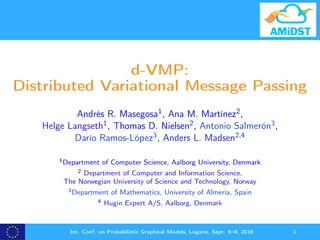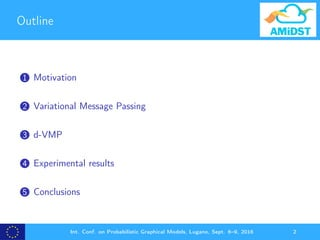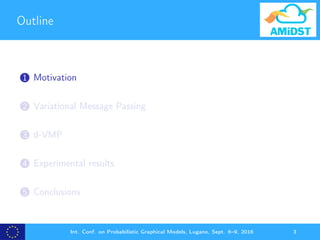Diese Präsentation wurde erfolgreich gemeldet.

# d-VMP: Distributed Variational Message Passing (PGM2016)

Anzeige
Anzeige
Anzeige
Anzeige
Anzeige
Anzeige
Anzeige
Anzeige
Anzeige
Anzeige
Anzeige×

1 von 36 Anzeige

# d-VMP: Distributed Variational Message Passing (PGM2016)

Motivated by a real-world financial dataset, we propose a distributed variational message passing scheme for learning conjugate exponential models. We show that the method can be seen as a projected natural gradient ascent algorithm, and it therefore has good convergence properties. This is supported experimentally, where we show that the approach is robust wrt. common problems like imbalanced data, heavy-tailed empirical distributions, and a high degree of missing values. The scheme is based on map-reduce operations, and utilizes the memory management of modern big data frameworks like Apache Flink to obtain a time-efficient and scalable implementation. The proposed algorithm compares favourably to stochastic variational inference both in terms of speed and quality of the learned models. For the scalability analysis, we evaluate our approach over a network with more than one billion nodes (and approx. 75% latent variables) using a computer cluster with 128 processing units.

Motivated by a real-world financial dataset, we propose a distributed variational message passing scheme for learning conjugate exponential models. We show that the method can be seen as a projected natural gradient ascent algorithm, and it therefore has good convergence properties. This is supported experimentally, where we show that the approach is robust wrt. common problems like imbalanced data, heavy-tailed empirical distributions, and a high degree of missing values. The scheme is based on map-reduce operations, and utilizes the memory management of modern big data frameworks like Apache Flink to obtain a time-efficient and scalable implementation. The proposed algorithm compares favourably to stochastic variational inference both in terms of speed and quality of the learned models. For the scalability analysis, we evaluate our approach over a network with more than one billion nodes (and approx. 75% latent variables) using a computer cluster with 128 processing units.

Anzeige
Anzeige

### d-VMP: Distributed Variational Message Passing (PGM2016)

1. 1. d-VMP: Distributed Variational Message Passing Andrés R. Masegosa1, Ana M. Martínez2, Helge Langseth1, Thomas D. Nielsen2, Antonio Salmerón3, Darío Ramos-López3, Anders L. Madsen2,4 1Department of Computer Science, Aalborg University, Denmark 2 Department of Computer and Information Science, The Norwegian University of Science and Technology, Norway 3Department of Mathematics, University of Almería, Spain 4 Hugin Expert A/S, Aalborg, Denmark Int. Conf. on Probabilistic Graphical Models, Lugano, Sept. 6–9, 2016 1
2. 2. Outline 1 Motivation 2 Variational Message Passing 3 d-VMP 4 Experimental results 5 Conclusions Int. Conf. on Probabilistic Graphical Models, Lugano, Sept. 6–9, 2016 2
3. 3. Outline 1 Motivation 2 Variational Message Passing 3 d-VMP 4 Experimental results 5 Conclusions Int. Conf. on Probabilistic Graphical Models, Lugano, Sept. 6–9, 2016 3
4. 4. Motivation Large Imbalance ? values Complex distributions Int. Conf. on Probabilistic Graphical Models, Lugano, Sept. 6–9, 2016 4
5. 5. Motivation Large Imbalance ? values Complex distributions Attribute range Density 0 1 2 3 4 5 6 0123456 ● ● ● ● SVI 1% SVI 5% SVI 10% VMP 1% Int. Conf. on Probabilistic Graphical Models, Lugano, Sept. 6–9, 2016 5
6. 6. Motivation Goal: learn a generative model for a ﬁnantial dataset to monitor the customers and make predictions for a single customer. Xi Hi θα i = 1, . . . , N Int. Conf. on Probabilistic Graphical Models, Lugano, Sept. 6–9, 2016 6
7. 7. Popular existing approach: SVI Stochastic Variational Inference: iteratively updates the model parameters based on subsampled data batches. No estimation of all local hidden variables of the model. No generation of lower bound. Poor ﬁt if batch of data is not representative from all data. Int. Conf. on Probabilistic Graphical Models, Lugano, Sept. 6–9, 2016 7
8. 8. Our contribution: d-VMP: a distributed message passing scheme. Deﬁned for a broader class of models (than SVI). Better and faster convergence results compared to SVI. Posterior over all local latent variables and lower bound. Int. Conf. on Probabilistic Graphical Models, Lugano, Sept. 6–9, 2016 8
9. 9. Outline 1 Motivation 2 Variational Message Passing 3 d-VMP 4 Experimental results 5 Conclusions Int. Conf. on Probabilistic Graphical Models, Lugano, Sept. 6–9, 2016 9
10. 10. Models: Bayesian learning on iid. data using conjugate exponential BN models: ln p(X) = ln hX + sX · η − AX (η) Xi Hi θα i = 1, . . . , N We want to calculate p(θ, H|D). Int. Conf. on Probabilistic Graphical Models, Lugano, Sept. 6–9, 2016 10
11. 11. Variational Inference: Approximate p(θ, H|D) (often intractable) by ﬁnding tractable posterior distributions q ∈ Q by minimizing: min q(θ,H)∈Q KL(q(θ, H)|p(θ, H|D)), Int. Conf. on Probabilistic Graphical Models, Lugano, Sept. 6–9, 2016 11
12. 12. Variational Inference: Approximate p(θ, H|D) (often intractable) by ﬁnding tractable posterior distributions q ∈ Q by minimizing: min q(θ,H)∈Q KL(q(θ, H)|p(θ, H|D)), In the mean ﬁeld variational approach, Q is assumed to fully factorize: q(θ, H) = M k=1 q(θk) N i=1 J j=1 q(Hi,j ), Int. Conf. on Probabilistic Graphical Models, Lugano, Sept. 6–9, 2016 12
13. 13. Variational Inference: Variational Inference exploits: ln P(D) constant = L(q(θ, H)) Maximize + KL(q(θ, H)|p(θ, H|D)) Minimize , Iterative coordinate ascent of the variational distributions. Updates in the variational distribution of a variable only involves variables in its Markov blanket. Coordinate ascent algorithm formulated as a message passing scheme. Int. Conf. on Probabilistic Graphical Models, Lugano, Sept. 6–9, 2016 13
14. 14. Variational Message Passing, VMP: Message from parent to child: moment parameters (expectation of the suﬃcient statistics). Message from child to parent: natural parameters (based on the messages received from the co-parents). Int. Conf. on Probabilistic Graphical Models, Lugano, Sept. 6–9, 2016 14
15. 15. Outline 1 Motivation 2 Variational Message Passing 3 d-VMP 4 Experimental results 5 Conclusions Int. Conf. on Probabilistic Graphical Models, Lugano, Sept. 6–9, 2016 15
16. 16. Distributed optimization of the lower bound: Master θα q(t)(θ) X H θ1 i = 1, . . . , N Slave 1 q(t)(H1) X H θ2 i = 1, . . . , N Slave 2 q(t)(H2) X H θ3 i = 1, . . . , N Slave 3 q(t)(H3) q(t)(θ) is broadcasted to all the slave nodes. maxq∈Q L(q(θ, H)) Int. Conf. on Probabilistic Graphical Models, Lugano, Sept. 6–9, 2016 16
17. 17. Distributed optimization of the lower bound: Master θα q(t)(θ) X H θ1 i = 1, . . . , N Slave 1 q(t)(H1) X H θ2 i = 1, . . . , N Slave 2 q(t)(H2) X H θ3 i = 1, . . . , N Slave 3 q(t)(H3) q(t+1)(H) = arg maxq(H) L(q(H), q(t)(θ)) maxq∈Q L(q(θ, H)) Int. Conf. on Probabilistic Graphical Models, Lugano, Sept. 6–9, 2016 17
18. 18. Distributed optimization of the lower bound: Master θα q(t)(θ) X H θ1 i = 1, . . . , N Slave 1 q(t)(H1) X H θ2 i = 1, . . . , N Slave 2 q(t)(H2) X H θ3 i = 1, . . . , N Slave 3 q(t)(H3) q(t+1)(Hn) = arg maxq(Hn) Ln(q(Hn), q(t)(θ)) maxq∈Q L(q(θ, H)) Int. Conf. on Probabilistic Graphical Models, Lugano, Sept. 6–9, 2016 18
19. 19. Distributed optimization of the lower bound: Master θα q(t)(θ) X H θ1 i = 1, . . . , N Slave 1 q(t)(H1) X H θ2 i = 1, . . . , N Slave 2 q(t)(H2) X H θ3 i = 1, . . . , N Slave 3 q(t)(H3) q(t+1) (θ) = arg max q(θ) L(q(t) (H), q(θ)) May be coupled maxq∈Q L(q(θ, H)) Int. Conf. on Probabilistic Graphical Models, Lugano, Sept. 6–9, 2016 19
20. 20. Candidate solutions: Resort to a generalized mean-ﬁeld approximation as SVI: does not factorize over the global parameters. Prohibitive for models with a large number of global (coupled) parameters, e.g. linear regression. Our proposal: VMP as a distributed projected natural gradient ascent algorithm (PGNA). Int. Conf. on Probabilistic Graphical Models, Lugano, Sept. 6–9, 2016 20
21. 21. d-VMP as a projected natural gradient ascent Insight 1: VMP can be expressed as a projected natural gradient ascent algorithm. η (t+1) X = η (t) X + ρX,t[ ˆ ηL(η(t) )]+ X (1) [·] is the projection operator. Int. Conf. on Probabilistic Graphical Models, Lugano, Sept. 6–9, 2016 21
22. 22. d-VMP as a projected natural gradient ascent Insight 2: The natural gradient of the lower bound can be expressed as follows: ˆ ηθ L = mPa(θ)→θ + mHi →θ The gradient can be computed in parallel. Int. Conf. on Probabilistic Graphical Models, Lugano, Sept. 6–9, 2016 22
23. 23. d-VMP as a projected natural gradient ascent Insight 3: Global parameters are “coupled” only if they belong to each other’s Markov blanket. Deﬁne a disjoint partition of the global parameters: R = {J1, . . . , JS } Int. Conf. on Probabilistic Graphical Models, Lugano, Sept. 6–9, 2016 23
24. 24. d-VMP as a projected natural gradient ascent d-VMP is based on performing independent global updates over the global parameters of each partition: η (t+1) Jr = η (t) Jr + ρr,t[ ˆ ηL(η(t) )]+ Jr ρr,t is the learning rate. If |Jr | = 1 then ρr,t = 1. Int. Conf. on Probabilistic Graphical Models, Lugano, Sept. 6–9, 2016 24
25. 25. dVMP as a distributed PNGA algorithm: Master θα η (t+1) Jr X H θ1 i = 1, . . . , N Slave 1 η (t+1) 1 X H θ2 i = 1, . . . , N Slave 2 η (t+1) 2 X H θ3 i = 1, . . . , N Slave 3 η (t+1) 3 η (t) Jr + ρr,t[ˆηL(η(t) )]+ Jr For all Jr ∈ R maxq∈Q L(q(θ, H)) Int. Conf. on Probabilistic Graphical Models, Lugano, Sept. 6–9, 2016 25
26. 26. Outline 1 Motivation 2 Variational Message Passing 3 d-VMP 4 Experimental results 5 Conclusions Int. Conf. on Probabilistic Graphical Models, Lugano, Sept. 6–9, 2016 26
27. 27. Model ﬁt to the data Xij HijYi Hi θ α j = 1, . . . , J i = 1, . . . , N Attribute range Density 0 1 2 3 4 5 6 0123456 ● ● ● ● SVI 1% SVI 5% SVI 10% VMP 1% Representative sample of 55K clients (N) and 33 attributes (J). “Unrolled” model of more than 3.5M nodes (75% latent variables). Int. Conf. on Probabilistic Graphical Models, Lugano, Sept. 6–9, 2016 27
28. 28. Model ﬁt to the data Int. Conf. on Probabilistic Graphical Models, Lugano, Sept. 6–9, 2016 28
29. 29. Model ﬁt to the data 0 500 1000 1500 2000 −1.5e+07−1.0e+07−5.0e+060.0e+00 Time (seconds) Globallowerbound Alg. BS(data%)/LR SVI 1%/0.55 SVI 1%/0.75 SVI 1%/0.99 SVI 5%/0.55 SVI 5%/0.75 SVI 5%/0.99 SVI 10%/0.55 SVI 10%/0.75 SVI 10%/0.99 d−VMP Int. Conf. on Probabilistic Graphical Models, Lugano, Sept. 6–9, 2016 29
30. 30. Test marginal log-likelihood BS (% data) LR Log-Likel. SVI 1 % 0.55 -180902.87 0.75 -298564.03 0.99 -426979.52 5 % 0.55 -177302.24 0.75 -333264.16 0.99 -628105.70 10 % 0.55 -347035.22 0.75 -397525.45 0.99 -538087.13 d-VMP 1.0 67265.34 Int. Conf. on Probabilistic Graphical Models, Lugano, Sept. 6–9, 2016 30
31. 31. Mixtures of learnt posteriors for one attribute ● ● ● ● SVI 1% SVI 5% SVI 10% VMP 1% Int. Conf. on Probabilistic Graphical Models, Lugano, Sept. 6–9, 2016 31
32. 32. Scalability settings Generated data set of 42 million samples per client and 12 variables. “Unrolled” model of more than 1 billion (109) nodes (75% latent variables). AMIDST Toolbox with Apache Flink. Amazon Web Services (AWS) as distributed computing environment. Int. Conf. on Probabilistic Graphical Models, Lugano, Sept. 6–9, 2016 32
33. 33. Scalability results Int. Conf. on Probabilistic Graphical Models, Lugano, Sept. 6–9, 2016 33
34. 34. Outline 1 Motivation 2 Variational Message Passing 3 d-VMP 4 Experimental results 5 Conclusions Int. Conf. on Probabilistic Graphical Models, Lugano, Sept. 6–9, 2016 34
35. 35. Conclusions Variational methods can be scaled using distributed computation instead of sampling techniques. Bayesian learning in model with more than 1 billion nodes (75% of hidden). Int. Conf. on Probabilistic Graphical Models, Lugano, Sept. 6–9, 2016 35
36. 36. Thank you for your attention Questions? You can download our open source Java toolbox: amidsttoolbox.com Acknowledgments: This project has received funding from the European Union’s Seventh Framework Programme for research, technological development and demonstration under grant agreement no 619209 Int. Conf. on Probabilistic Graphical Models, Lugano, Sept. 6–9, 2016 36